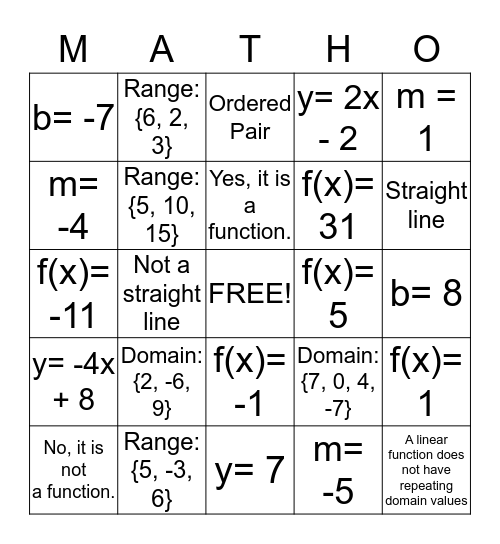# Domain, Range, Functions, & SlopeThis bingo card has a free space and 24 words: Domain: {2, -6, 9}, Range: {5, -3, 6}, Yes, it is a function., No, it is not a function., m = 1, b= -7, m= -4, Range: {6, 2, 3}, f(x)= 5, f(x)= -1, f(x)= 31, f(x)= -11, Domain: {7, 0, 4, -7}, f(x)= 1, Range: {5, 10, 15}, m= -5, b= 8, Ordered Pair, Straight line, Not a straight line, A linear function does not have repeating domain values, y= -4x + 8, y= 2x - 2 and y= 7.

## Play Online

Share this URL with your players:For more control of your online game, create a clone of this card first.

## Probabilities

With players vying for a you'll have to call about __ items before someone wins. There's a __% chance that a lucky player would win after calling __ items.

Tip: If you want your game to last longer (on average), add more unique words/images to it.